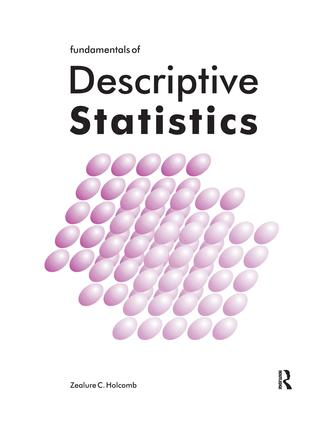Fundamentals of Descriptive Statistics

1st Edition

Routledge

98 pages

Purchasing Options:\$ = USD
Paperback: 9781884585050
pub: 1997-09-01
SAVE ~\$7.99
\$39.95
\$31.96
x
eBook (VitalSource) : 9781315266510
pub: 2016-09-13
from \$19.98

FREE Standard Shipping!

Description

• Do your students need to organize and summarize data for term projects? Will they need to perform these tasks on the job? This book gives them thorough preparation.

• In twelve short chapters, your students will learn the purposes of descriptive statistics, their calculation, and proper interpretation.

• Actual data on the emotional health of foster-care adolescents are used throughout the book to illustrate various ways of deriving meaning from the data with descriptive statistics. Other interesting examples are also included.

• Computational procedures are illustrated with step-by-step, easy-to-follow examples.

• End-of-chapter exercises provide ample practice for students to master both computations and statistical concepts.

• Eliminates the need for students to buy a traditional statistics book that emphasizes inferential statistics.

• Thoroughly field-tested for student comprehension.

• This book will please you and your students with its clarity of presentation.

• Outstanding supplement for students who need to describe term project data.

Chapter 1: Introduction to Statistics

Chapter 2: Percentages and Bar Graphs

Chapter 3: Shapes of Distributions

Chapter 4: The Mean: The Most Popular Average

Chapter 5: The Median and Mode: Alternatives to the Mean

Chapter 6: The Standard Deviation: First Cousin of the Mean

Chapter 7: The Range, Quartiles, and Interquartile Range

Chapter 8: Standard Scores

Chapter 9: Scattergrams and the Pearson r

Chapter 10: The Coefficient of Determination

Chapter 11: Linear Regression

Chapter 12: Glossary of Descriptive Statistics

Appendix A: Formula for the Interpolated Median

Appendix B: Computational Formula for the Standard Deviation

Appendix C: Computational Formula for the Pearson r

Index